## Current Issues

### Numerical Simulation of Inertia Matrix for 6 DoF Industrial Robot

1 Department of Robotics , Lola Institut , Serbia

2 Department of Robotics, Mihajlo Pupin Institute , University of Belgrade , Serbia

J Robot Mech Eng

Article Type : Research Article

### Abstract

The robot dynamic model is essential for the precision and reliability of robot design, motion control, and simulation. A robot inertia matrix, whose elements are coefficients of joint accelerations within the robot equations of motion, plays an important role in the robot’s control design. During robot motion, elements of the inertia matrix are functions of robot configuration (robot joint positions). To facilitate the development of process models and to make an appropriate selection of motion control algorithms, it is useful to perform numerical simulations of inertia matrix elements for different robot trajectories. In this paper, numerical simulation of inertia matrix is presented for 6 DoF industrial robot with revolute joints for the programmed robot motion. Inertia matrix is obtained from the robot dynamical model developed by using modified recursive Newton-Euler algorithm. Based on the presented simulations, variation of effective inertias and magnitude and variation of cross-coupling effects in the robot inertia matrix are examined.

Keywords: Inertia Matrix; Mass Matrix; Robot; Simulation

# Introduction

Dynamics play a fundamental role in motion simulation, manipulation structure analysis, and control algorithm synthesis of robots [1-3]. The past several decades saw a considerable amount of research regarding the computational efficiency of robot dynamic models for various robots and multibody systems . Owing to the development of modern-day computers, the ease of development and implementation (algorithm development for a specific robot) of an algorithm is another highly important feature, arguably even more important than computational complexity . Recursive methods are the most computationally efficient methods for the development of the robot equations of motion, e. g. recursive Newton-Euler algorithm (RNEA) is the most efficient computational method used for deriving a robot’s dynamic equations of motion . However, by using other methods such as Lagrange formulation of manipulator equations of motion, a compact analytical form containing n×n generalized robot mass (inertia) matrix H(q), and a bias vector u’, that denotes joint torque contributions which do not correlate with the joint accelerations, are obtained. The dynamic model in a compact form is important because it is used to design controllers and to analyze the stability, in the sense of Lyapunov, of the equilibria of the closed-loop system . There are various ways to simplify the dynamic equations of a particular robot manipulator for control purposes . One way is through the joint-space inertia matrix, which maps the joint accelerations to the joint torques/forces. The properties of the robot inertia matrix are comprehensively used in control design for robots . Inertia matrix is symmetric, non–singular, and positive definite for each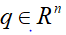. For revolute joint robots, the inertia matrix also satisfies global bounds that are useful for control design . In single joint control methods, diagonal elements of the inertia matrix influence the pole placement region of a closed-loop control system of a robot . Each element of an inertia matrix is a relatively complex expression of joint positions that depends on the numerical values of robot parameters such as masses, inertias, and positions of centers of masses. It is argued that the general physical interpretation of the robot inertia matrix in which the diagonal terms are related to the effective inertias of the corresponding DoFs, and the off-diagonal terms express the inertial couplings between the DoFs is somewhat insufficient .

The inertia matrix can be simplified so that it accounts for the major coupling between axes but not for minor cross-coupling effects [5, 10]. This can achieve a significant reduction in the number of calculations, and more importantly, it could facilitate the practical process of control system design. There are not many papers in the literature where numerical calculation of real robot manipulator inertia matrix elements is given. To examine cross-coupling effects of non-diagonal inertia matrix elements for a desired robot trajectory and the magnitude of variation of diagonal elements, numerical simulations of robot inertia matrix elements can be used within design of control algorithms.

In this paper, the numerical simulation of inertia matrix members for the programmed motion of 6DoF industrial robot RL15 is presented. Inertia matrix in closed form is obtained by implementation of the novel modified recursive Newton-Euler algorithm (mRNEA) presented in .

# Calculation of Inertia Matrix Based on Mrnea for 6DoF Robot RL15

Motion equation for a manipulator with n degrees of freedom can be described in the following matrix form: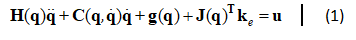where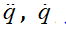and q are n×1 vectors of the joint accelerations, velocities, and positions, respectively; is an n×n matrix consisting of centrifugal and Coriolis terms; g(q) is an n×1 vector of gravity terms; ke is a 6×1 vector of the external forces and moments acting on the last link n; J(q) is a 6×n Jacobian matrix; and u is an n×1 vector of the input joint torques/forces.

The n×n matrix H(q) in Eq. (1) is known in the literature as the inertia matrix of a robot. When the inertia matrix is diagonal (no inertial coupling between any of the joint accelerations), it is obvious that each diagonal term represents the effective inertia associated with the corresponding joint acceleration. If the mass matrix is not diagonal, there is coupling between the generalized accelerations, and a torque/force applied by the motor in one robot joint will cause acceleration in the coupled joints. The coupling magnitude depends on the robot configuration, while the overall significance of the diagonal terms is no longer clear . For the development of the mass matrix, several algorithms are presented in the literature [11-15]. In this paper, mRNEA algorithm is used to obtain equations of motion for the 6DoF robot RL15 from which inertia matrix elements are obtained using recursion . The robot dynamic model is discretized in order to be suitable for implementation within discrete simulation systems and robot controllers , and for each interpolation period, it is given in the following form: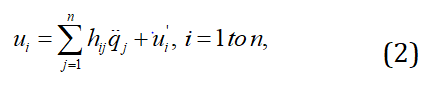Given that motors with gearboxes are implemented in robot links, it is suitable to develop robot equations of motion relative to robot actuators, Eq. (3) :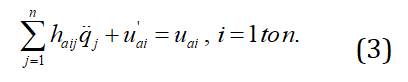Where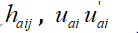relate to actuator rotors. Gear ratios for actuators of RL15 are given in . In presented simulations, friction is neglected.

# Description of 6DoF robot RL15

RL15, Figure 1, is a 6DoF industrial robot with revolute joints, which is used for the development of experiments related to the development of motion control system of industrial robots. Calculation of inertial parameters of robot links (links’ masses and the moments of inertia about coordinate frame attached to the links) and geometric parameters of robot links, such as mass center coordinates etc., was performed in CATIA software. The moments of inertia around the x, y and z axes of the coordinate frames attached to the robot links, respectively and the masses of the RL15 links are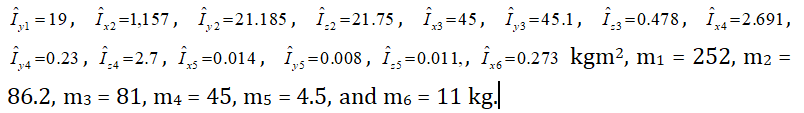The viscous and static coefficients of friction are neglected in the presented simulations. Effects of geometric (kinematic) coupling in the movement of the robot links, gearbox ratios, and data on implemented actuators are given in . Axes 1 and 3 of the RL15 are actuated with the motors with maximum torque of 17.8 Nm. The maximum number of revolutions is 3150

min-1. Axis 2 has a motor with a maximum torque of 38.6 Nm and the maximum number of revolutions of 2800 min-1. Axes 4, 5, and 6 have motors with the maximum torque of 3.47 Nm and the maximum number of revolutions of 3400 min-1. Within the simulation program, the external forces are fex=100, fey=100, and fez=–150 N.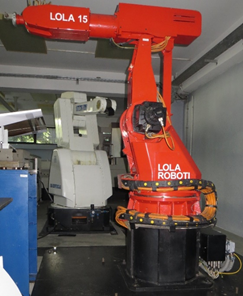Figure 1: 6DoF robot RL15.

# Results and Discussion

Numerical simulations of the inertia matrix elements are performed for the trajectory of the end-effector of robot RL15, which is programmed in L-IRL robot programming language [17-18], and is presented in Figure 2a. The motion of RL15 in Figure 2a is visualized in the previously developed system for simulation and supervision of robotic cells . For the end-effector motion given in Figure 2a, joint trajectories obtained from the RL15 trajectory planner are given in Figure 2b. Interpolation period is 5ms. For the trajectory given in Figure 2b, inertia matrix elements relative to robot actuators, i.e. coefficients haij, i=1..6, j=1..6, obtained using Eq. (3) with haij=hij/ri (ri is the gear ratio of the gearbox of axis i, note that in Eq. (3) acceleration of robot link, not rotor’s shaft appears) are calculated. In Figure 3, variation of selected elements haij of inertia matrix is given. In Table 1, maximal absolute values of inertia matrix elements relative to manipulator actuators haij for the joint trajectory given in Figure 2b are given (the values of diagonal inertia matrix elements are always positive).

As it can be seen, angular acceleration coefficients hij in robot dynamic model are bounded. Fast variations present within several haij coefficients appear due to highly complex trigonometric dependence on robot joint positions. From numerical simulations presented in Figure 3, we can conclude that for the programmed robot motion of robot RL15 given in Figures 2, effective inertia (diagonal elements in matrix H(q)) vary significantly for axes 1-2, somewhat for axes 3-4, and negligibly for axes 5-6. This fact, together with the ratios of diagonal and non-diagonal coefficients hij could be used to simplify inertia matrix H(q), i.e. robot model, depending on the choice of the control strategy. Further research is necessary in this direction.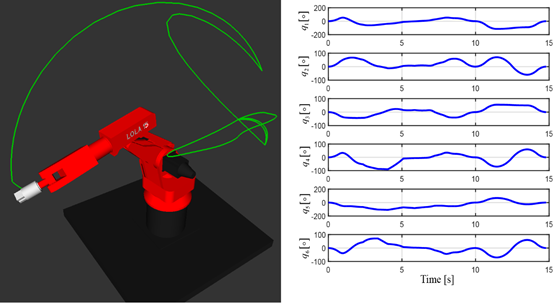Figure 2a:Programmed end-effector motion of 6DoF robot RL15.
Figure 2b: Joint motions of robot RL15 for the end-effector trajectory presented in Figure 2a.

 i hai1max hai2max hai3max hai4max hai5max hai6max 1 3.07 0.03 0.03 0.028 0.03 1.3?10-4 2 0.27 20.2 16.3 0.28 0.35 0.01 3 0.02 1.01 1.48 0.16 0.02 1.3?10-4 4 0.03 0.02 0.03 0.12 6.4?10-6 1.2?10-4 5 0.02 0.018 0.02 0.002 0.22 ?0 6 3?10-4 3?10-4 3?10-4 1?10-4 0.00373 0.0376

Table 1: Maximal absolutevalues of inertia matrix elements haij relative to manipulatoractuators for RL15 for the joint trajectory given in Figure 2.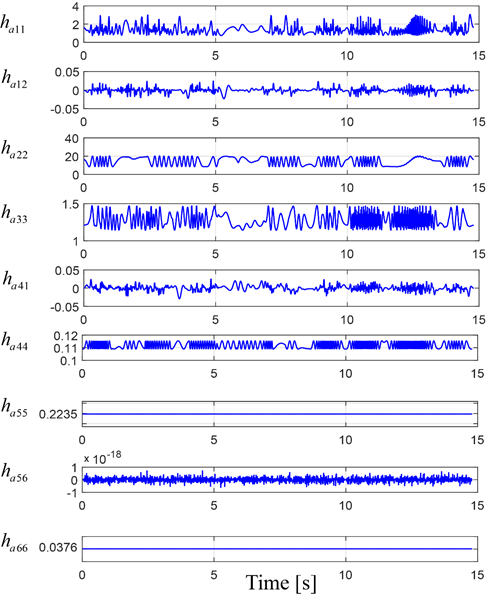Figure 3: Variation of selectedinertia matrix elements haij=hij/ri for RL15joint trajectories presented in Figure 2b.

# Conclusion

In this paper, numerical simulation of robot inertia matrix is presented for 6 DoF industrial robot for the desired robot motion. Simulation of inertia matrix elements is based on the discretized dynamic model of the robot obtained using the modified recursive Newton-Euler algorithm. The obtained graphs are intended to be used for control design purpose to determine the variation of effective inertias and magnitude and variation of cross-coupling effects in the robot inertia matrix. Further research should be carried out in order to examine the possibilities of simplification of robot inertia matrix for motion control design purposes.

# Acknowledgements

This research has been supported by the research grants of the Serbian Ministry of Education, Science and Technological Development.

# References

1. Kvrgic V, Vidakovic J. Efficient Method for Robot Forward Dynamics Computation. Mech Mach Theory. 2020;145:103680.

2. Jubien A, Gautier M, Janot A. Dynamic Identification of the Kuka Lightweight Robot: Comparison between Actual and Confidential Kuka's Parameters. 2014 IEEE/ASME International Conference on Advanced Intelligent Mechatronics. 2014;483-488.

3. Sciavicco L, Siciliano B. Modelling and Control of Robot Manipulators. Springer Science & Business Media. 2012.

4. Kelly R, Davila VS, Perez JAL. Control of Robot Manipulators in Joint Space. Springer Science & Business Media. 2006.

5. Craig JJ. Introduction to Robotics: Mechanics and Control. (3rd ed), Pearson Prentice Hall, Upper Saddle River. 2005.

6. Siciliano B, Khatib O. Springer Handbook of Robotics. Springer. 2016.

7. Hirschkorn M, Kövecses J. The Role of the Mass Matrix in the Analysis of Mechanical Systems. Multibody Sys.Dyn 2013;30:397-412.

8. Spong Mark W, Seth Hutchinson, Mathukumalli Vidyasagar. Robot modeling and control. 2006;3.

9. Vidakovi? J, Kvrgi? V, Lazarevi? M, et al. Computed Torque Control for a Spatial Disorientation Trainer. Facta Universitatis, Series: Mechanical Engineering. 2020;18:269-280.

10. Armstrong B, Khatib O, Burdick J. The Explicit Dynamic Model and Inertial Parameters of the PUMA 560 Arm. 1986 IEEE international conference on robotics and automation. 1986;3:510-518.

11. Featherstone R. The Calculation of Robot Dynamics Using Articulated–Body Inertias Position and Velocity Transformations between Robot End Effector Coordinates and Joint Angles. Int J Rob Res. 1983;2:13–30.

12. Drumwright E. Fast Dynamic Simulation of Highly Articulated Robots with Contact via O(n2) Time Dense Generalized Inertia Matrix Inversion, in: Simulation, Modeling, and Programming for Autonomous Robots, Noda I, Ando N, Brugali D, et al (Eds.), SIMPAR 2012, Lecture Notes in Computer Science, Springer, Berlin, Heidelberg. 2012;7628:65–76.

13. Mata V, Provenzano S, Valero F, et al. Serial–Robot Dynamics Algorithms for Moderately Large Numbers of Joints. Mech Mach Theory. 2002;37:739–755.

15. Kvrgic V, Vidakovic ?. Calculation of Achievable Robot Joint Accelerations Based on a New Robot Forward Dynamics Algorithm. 7th International Conference on Electrical, Electronic and Computing Engineering IcETRAN 2020. 2020;28-30.

16. Mitrovi? S, Dimi? Z, Vidakovi? J, et al. System for Simulation and Supervision of Robotic Cells. In 12th International Scientific Conference on flexibile technologies Mma. 2015.

17. Lutovac M, Ferenc G, Kvrgic V, et al. Robot programming system based on L-IRL programming language. Acta Technica Corviniensis-Bulletin of Engineering. 2012;5:27.

18. Lutovac M, Ferenc G, Vidakovi? J, et al. Usage of XML and P Code for Robot Motion Control. In 2012 Mediterranean Conference on Embedded Computing (MECO). 2012:162-165.